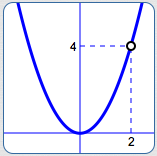﻿ Introduction to Puncture Points (holes)

# INTRODUCTION TO PUNCTURE POINTS (HOLES)

• PRACTICE (online exercises and printable worksheets)

Rational functions can exhibit puncture points (also called ‘holes’).

As you'll learn in Calculus, puncture points are an example of a ‘removable discontinuity’.Here's the idea.

The function $\displaystyle R(x) := \frac{x^3-2x^2}{x-2}\,$ certainly looks like a typical rational function.
Upon closer inspection, though, we see that there's an extra factor of $\,1\,$ in the formula: $$\frac{x^3 - 2x^2}{x - 2} \quad = \quad \frac{x^2(x-2)}{x-2} \quad = \quad x^2\cdot\frac{x-2}{x-2}$$ Therefore, $\,\displaystyle R(x) = \frac{x^3-2x^2}{x-2}\,$ has exactly the same outputs as the much simpler function, $\,P(x) := x^2\,$,
except that the function $\,R\,$ it isn't defined when $\,x = 2\,$.

The graphs of both $\,P\,$ and $\,R\,$ are shown below—the puncture point (hole) in $\,R\,$ is caused by that extra factor of $\,1\,$.
 $P(x) = x^2$$\displaystyle R(x) = \frac{x^3 - 2x^2}{x-2} = x^2\cdot\frac{x-2}{x-2}$Puncture points are studied in more detail in a future section.

Master the ideas from this section